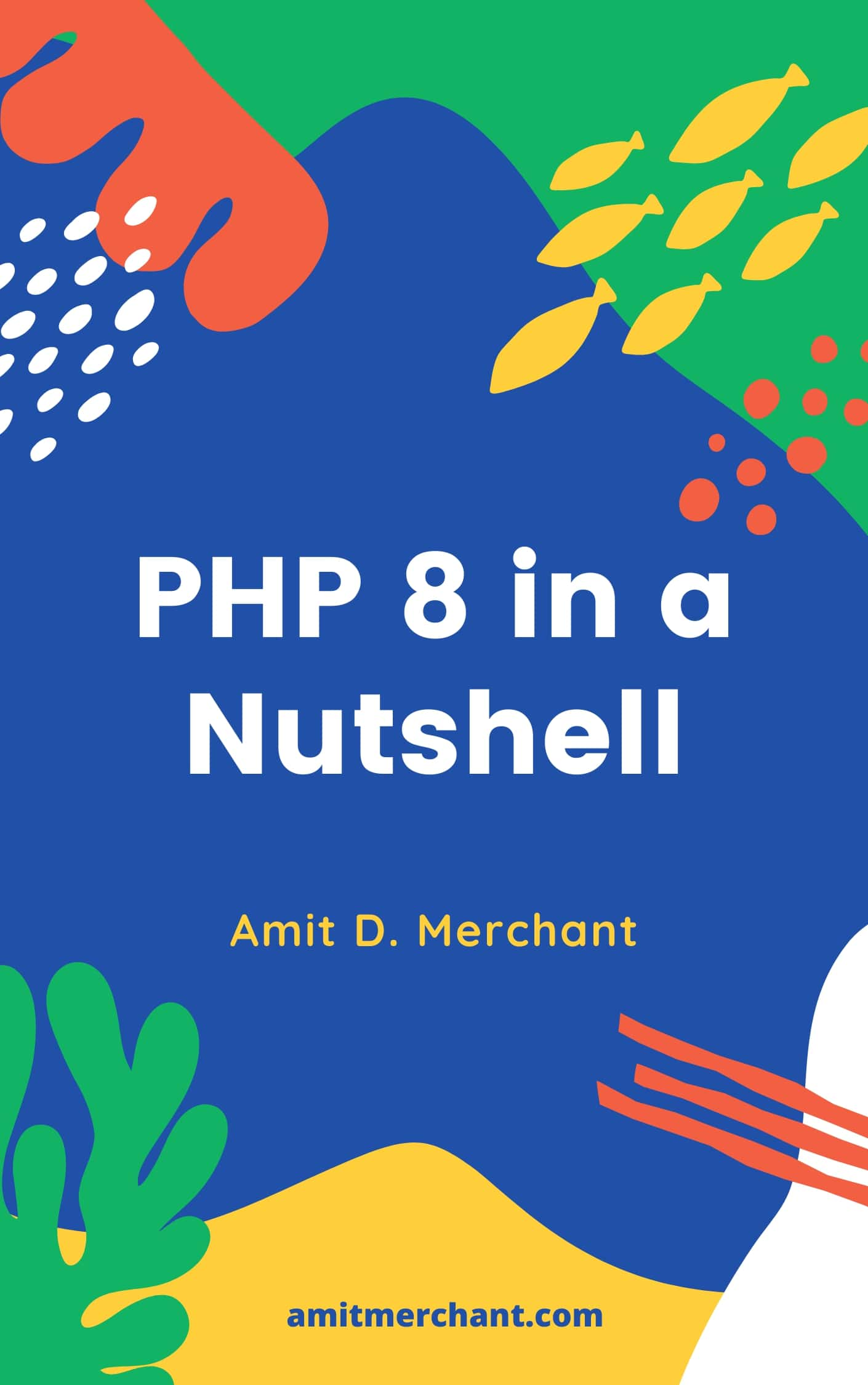# Amit MerchantVerified (\$10/year for the domain)

A blog on PHP, JavaScript, and more

# How to implement wrapper classes in PHP

If you look at PHP’s core helper functions, they aren’t very intuitive to use and are pretty inconsistent at times. For instance, the array_map function is used to apply the callback to the elements of the given arrays. Here’s how its definition looks like.

``````array_map ( callable \$callback , array \$array1 [, array \$... ] ) : array
``````

It accepts two parameters. The first one is the callback/closure which gets mapped on all the elements of the array which gets passed as a second argument.

And on the other hand, there’s this other function array_filter which can be used to filter elements of an array using a callback function. Here’s how its definition looks like.

``````array_filter ( array \$array [, callable \$callback [, int \$flag = 0 ]] ) : array
``````

Notice, how both the functions have parameter inconsistency. The first one have the callback as the first argument whereas the second one has it as the second argument.

Consistency like this makes the developer want to look at the documentation just to make sure the parameter sequence. This is ultimately leads to less efficiency while development.

This is where wrapper classes comes into play. A wrapper class is a class which makes the underlying functionality easier to use in some circumstances. Using wrapper classes, one can make the inconsistent functionalities consistent by using fluent APIs which acts as a wrapper for these functions/methods and this is what I’m going to cover in this article.

## Implementation

We’ll first create a class called `ArrayUtils` (which by the way is our wrapper class) which will hold all the array utilities and define a `getInstance` static method whose role is to just return the class instance.

``````<?php

class ArrayUtils
{
public static function getInstance()
{
return new ArrayUtils();
}
}
``````

Fair enough! Next, we’ll need a function to collect the array. So, we can define a public method called `collect` like so.

``````<?php

class ArrayUtils
{
private \$collection;

public static function getInstance()
{
return new ArrayUtils();
}

public function collect(array \$collection)
{
\$this->collection = \$collection;

return \$this;
}
}
``````

As you can see, the `collect` method accepts an argument of type `array` as its only argument and assign it to the private property `\$collection` and returns the object instance by returning `\$this`. This is important because we’re using method chaining to pass the array to the next method which I’m going to show next.

Now, all we need to define the method which encapsulates the PHP core method under the hood. Let’s make one for `array_map`.

``````<?php

class ArrayUtils
{
private \$collection;

public static function getInstance()
{
return new ArrayUtils();
}

public function collect(array \$collection)
{
\$this->collection = \$collection;

return \$this;
}

public function map(\Closure \$closure)
{
return array_map(\$closure, \$this->collection);
}
}
``````

As you can see, I’ve written a `map` method which accepts a closure/callback as it’s only arguments. We pass it to the `array_map`’s first argument and because we’re using fluent API, we’re also able to access `\$this->collection` which we’ve passed as a second argument.

So, that’s it! That’s all we needed to implement the wrapper function around PHP’s `array_map`.

## Usage

Now, let’s take a look at how you can use this class.

``````\$mappedArray = ArrayUtils::getInstance()
->collect([1, 2, 3, 4, 800])
->map(function(\$iteration) {
return \$iteration * 2;
});

print_r(\$mappedArray);

/*Array
(
 => 2
 => 4
 => 6
 => 8
 => 1600
)*/
``````

As you can see, we called `getInstance` static method on the `ArrayUtils` class, and the chained `collect` onto it passing in the input array. And we finally called `map` where we passed the callback using which the array will get mapped.

Similarly, we can implement an another method `filter` which can be synonymous to `array_filter` like so.

``````public function filter(\Closure \$closure)
{
return array_filter(\$this->collection, \$closure);
}
``````

And this is how you can utilize it.

``````\$filteredArray = ArrayUtils::getInstance()
->collect([1, 2, 3, 4, 800])
->filter(function(\$iteration) {
return \$iteration & 1; // Filter odd numbers
});

print_r(\$filteredArray);

/*Array
(
 => 1
 => 3
)
*/
``````

As you can see, the methods now looks more readable and as both of the methods follow a similar pattern, it’s now easier to remember the syntax. Similarly, we can implement other array methods this way, like the ones I’ve implemented below.

``````public function contains(\$item): bool
{
return in_array(\$item, \$this->collection);
}

public function getValues()
{
return array_values(\$this->collection);
}

public function getKeys()
{
return array_keys(\$this->collection);
}

public function search(string \$searchParam)
{
return array_search(\$searchParam, \$this->collection);
}
``````

I’ve created a repository where I’ve implemented all these examples which you run on the CLI as instructed on its readme. Take a look and if there are any doubts, let me know in the comments below.

👋 Hi there! I'm Amit. I write articles about all things web development. If you like what I do and want me to continue doing the same, I'd like you consider leaving a tip. I'd highly appreciate that. Cheers!(Includes PHP 8.1 and 8.2)

My Tiny YouTube Channel ➔